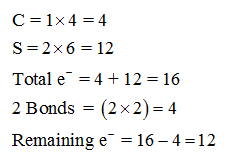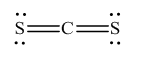# Which of the following will have a Lewis structure most like that of carbon disulfide? Group of answer choicesa. NO2-b. SO2c. waterd. NO2+e. CH4

Question
56 views
Which of the following will have a Lewis structure most like that of carbon disulfide?

a. NO2-
b. SO2
c. water
d. NO2+
e. CH4
check_circle

Step 1

Lewis structure can be drawn by the following steps,

The Lewis electron dot structure for given molecules are determined by first drawing the skeletal structure for the given molecules, then the total number of valence electrons for all atoms present in the molecules are determined.

The next step is to subtract the electrons present in the total number of bonds present in the skeletal structure of the molecule with the total valence electrons such that considering each bond contains two electrons with it.

Finally, the electrons which got after subtractions have to be equally distributed considering each atom contains eight electrons in its valence shell.

Step 2

Lewis structure of carbon disulfide is shown below,

Outer valence electrons of Carbon and sulfur are 4 and 6 respectively.Since octet rule does not obey for all the atoms, a double bond is made between carbon and sulfur atoms. Therefore, Lewis structure of carbon disulfide follows as,...

### Want to see the full answer?

See Solution

#### Want to see this answer and more?

Solutions are written by subject experts who are available 24/7. Questions are typically answered within 1 hour.*

See Solution
*Response times may vary by subject and question.
Tagged in